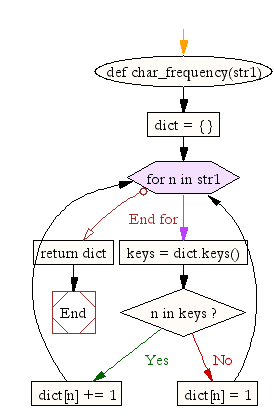﻿ Python: Count the number of characters (character frequency) in a string - w3resource# Python: Count the number of characters (character frequency) in a string

## Python String: Exercise-2 with Solution

Write a Python program to count the number of characters (character frequency) in a string.Sample Solution:-

Python Code:

``````def char_frequency(str1):
dict = {}
for n in str1:
keys = dict.keys()
if n in keys:
dict[n] += 1
else:
dict[n] = 1
return dict
```
```

Sample Output:

```{'g': 2, 'o': 3, 'l': 1, 'e': 1, '.': 1, 'c': 1, 'm': 1}
```

Flowchart:## Visualize Python code execution:

The following tool visualize what the computer is doing step-by-step as it executes the said program:

Python Code Editor:

Have another way to solve this solution? Contribute your code (and comments) through Disqus.

What is the difficulty level of this exercise?

Test your Programming skills with w3resource's quiz.

﻿

## Python: Tips of the Day

Check if a given key already exists in a dictionary:

In is the intended way to test for the existence of a key in a dict.

```d = {"key1": 10, "key2": 23}

if "key1" in d:
print("this will execute")

if "nonexistent key" in d:
print("this will not")
```

If you wanted a default, you can always use dict.get():

```d = dict()

for i in range(100):
key = i % 10
d[key] = d.get(key, 0) + 1
```

and if you wanted to always ensure a default value for any key you can either use dict.setdefault() repeatedly or defaultdict from the collections module, like so:

```from collections import defaultdict

d = defaultdict(int)

for i in range(100):
d[i % 10] += 1
```

but in general, the in keyword is the best way to do it.

Ref: https://bit.ly/2XPMRyz